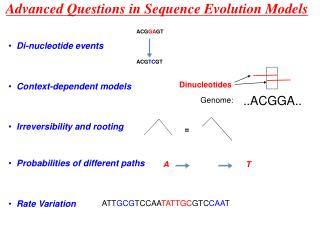DownloadDownload PresentationAdvanced Questions in Sequence Evolution Models

# Advanced Questions in Sequence Evolution Models

Download Presentation## Advanced Questions in Sequence Evolution Models

- - - - - - - - - - - - - - - - - - - - - - - - - - - E N D - - - - - - - - - - - - - - - - - - - - - - - - - - -
##### Presentation Transcript

1. Advanced Questions in Sequence Evolution Models ACGGAGT • Di-nucleotide events ACGTCGT Dinucleotides • Context-dependent models ..ACGGA.. Genome: • Irreversibility and rooting = • Probabilities of different paths A T • Rate Variation ATTGCGTCCAATATTGCGTCCAAT

2. Di-nucleotide events ACGGAGT ACGGAGT The Problem: ACGGAGT ACGTCGT ACGTCGT = ? Double events Single nucleotide events Data: ACGTCGT Averof et al. (2000) Evidence for High Frequency of Simultaneous Double-Nucleotide Substitutions” Science287.1283- . + Smith et al. (2003) A Low rate of Simultaneous Double-Nucleotide Mutations in Primates” Mol.Biol.Evol 20.1.47-53 Doublet Singlet Singlet Analysis and Conclusion: Assuming JC69 + doublet mutations. 00: 10-8 doublet mutation rate , ~10% of singlet rate 03: much less for a large more reliable data set

3. From singlet models to doublet models: Contagious Dependence: Independence Independence with CG avoidance Strand symmetry Only single events Single events with simple double events Pedersen and Jensen, 2001 Siepel and Haussler, 2003 Context-dependent models The Problem: What is P[CA]? G A C ? A C A T

4. The Gibbs Sampler Target Distribution is Both random & systematic scan algorithms leaves the true distribution invariant. The conditional distributions are then: An example: x2 x1 The approximating distribution after t steps of a systematic GS will be: For i=1,..,d: Draw xi(t+1) from conditional distribution p(.|x[-i](t)) and leave remaining components unchanged, i.e. x[-i] (t+1) = x[-i](t)

5. The Data: 100 kb non-coding from chromosomes 22 and 10 from mouse and human. From Lunter & Hein,2004 Basic Dinucleotide model • Jensen-Pedersen sampler (2000) sampler Sampling Sampled Sampled

6. Rooting using irreversibility (Lunter) General rate mode for nucleotides - 12 parameters: A C G T A qA,C qA,G qA,T C qC,A qC, G qC ,T G qG,A qG,C qG,T T qT,A qT,C qT,G Reversibility The Pulley Principle P( )= P( )* P( )* P( ) = = Reversible rate matrix : piqi,j=pjqj,j 9 parameters Felsenstein 1981 = Irreversibility used for rooting Ziheng Yang 1994

7. Irreversibility and rooting Inferred root positions: chr 21 .484 -/+.014 chr 10 .510 -/+ .016 Inferred position 0.33-/+0.03, true position 0.3

8. positions 1 n 1 sequences k slow - rs HMM: fast - rf Likelihood Recursions: Likelihood Initialisations: Fast/Slowly Evolving States Felsenstein & Churchill, 1996 • pr - equilibrium distribution of hidden states (rates) at first position • pi,j - transition probabilities between hidden states • L(j,r) - likelihood for j’th column given rate r. • L(j,r) - likelihood for first j columns given j’th column has rate r.

9. Fast-Slow HMM Application

10. Probabilities of different paths A T • What are the number of events? • What are the kinds of events?

11. Geometric/Exponential Distributions The Geometric Distribution: {1,..} Geo(p): P{Z=j)=pj(1-p) P{Z>j)=pj E(Z)=1/p. The Exponential Distribution: R+ Exp (a) Density: f(t) = ae-at, P(X>t)= e-at Properties: X~Exp(a) Y~Exp(b) independent i. P(X>t2|X>t1) = P(X>t2-t1) (t2 > t1) ii. E(X) = 1/a. iii. P(Z>t)=(≈)P(X>t) small a (p=e-a). iv. P(X < Y) = a/(a + b). v. min(X,Y) ~ Exp (a + b).